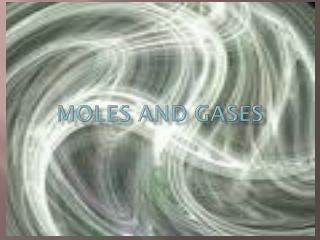# MOLES AND GASES - PowerPoint PPT PresentationDownload PresentationMOLES AND GASES

MOLES AND GASESDownload Presentation## MOLES AND GASES

- - - - - - - - - - - - - - - - - - - - - - - - - - - E N D - - - - - - - - - - - - - - - - - - - - - - - - - - -
##### Presentation Transcript

1. MOLES AND GASES

2. Molar Volume 1 mole of any gas at (STP) standard temperature (0C)andpressure (1 atm) has a volume of 22.4 cubic decimeters (dm3), which is the same as 22.4 liters (L). This volume of gas is called a molar volume because it is the volume of 1 mole of a gas at standard conditions.

3. Moles Volume (L) To calculate molar volume of a gas: (X) Moles22.4 L/mol = Molar volume (L) 1 mol

4. Volume (L)  Moles To calculate moles: (÷) Molar volume of gas 1 mol = Moles 22.4 L/mol

5. Sample A: VOLUME (L)  MOLES (÷) A room with a volume of 4000 L contains how many moles of air at STP?

6. Sample B: MOLES  VOLUME (L) (X) A chemical reaction produces 0.82 moles of oxygen gas. What volume will that gas occupy at STP?

7. PRACTICE PROBLEMS 1.) A container with a volume of 893 L contains how many moles of air at STP? (L  mol)

8. 2.) A chemical reaction produces 0.37 mol of N2gas. What volume will that gas occupy at STP? (mol  L)

9. Convert grams  liters(g  mol  L) 6. 5.0g of H2

10. 7. 100g of O2